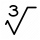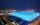# Square root + third power - math problems

#### Number of problems found: 20

• One third powerWhich equation justifies why ten to the one-third power equals the cube root of ten?
• Derivative problemThe sum of two numbers is 12. Find these numbers if: a) The sum of their third powers is minimal. b) The product of one with the cube of the other is maximal. c) Both are positive and the product of one with the other power of the other is maximal.
• Cube 6Surface area of one wall cube is 1600 cm square. How many liters of water can fit into the cube?
• Sphere A2VThe surface of the sphere is 241 mm2. What is its volume?
• Rectangle poolDetermine dimensions of open pool with a square bottom with a capacity 32 m3 to have painted/bricked walls with least amount of material.
• Cube-shaped boxThe cube-shaped box is filled to the brim with 2 liters of milk. Calculate the edge and surface of the box.
• Cube diagonalsCalculate the length of the side and the diagonals of the cube with a volume of 27 cm3.
• Alien shipThe alien ship has the shape of a sphere with a radius of r = 3000m, and its crew needs the ship to carry the collected research material in a cuboid box with a square base. Determine the length of the base and (and height h) so that the box has the large
• Cube V2SThe volume of the cube is 27 dm cubic. Calculate the surface of the cube.
• Cube surfce2volumeCalculate the volume of the cube if its surface is 150 cm2.
• Three members GPThe sum of three numbers in GP (geometric progression) is 21 and the sum of their squares is 189. Find the numbers.
• Volume and areaWhat is the volume of a cube which has area of 361 cm2?
• Length of the edgeFind the length of the edge of a cube that has a cm2 surface and a volume in cm3 expressed by the same number.
• SphereThe surface of the sphere is 12100 cm2, and the weight is 136 kg. What is its density?
• Body diagonalThe cuboid has a volume of 32 cm3. Its side surface area is double as one of the square bases. What is the length of the body diagonal?
• Pyramid cutWe cut the regular square pyramid with a parallel plane to the two parts (see figure). The volume of the smaller pyramid is 20% of the volume of the original one. The bottom of the base of the smaller pyramid has a content of 10 cm2. Find the area of the
• BallsThree metal balls with volumes V1=71 cm3 V2=78 cm3 and V3=64 cm3 melted into one ball. Determine it's surface area.
• Cuboid to cubeA cuboid with dimensions of 9 cm, 6 cm, and 4 cm has the same volume as a cube. Calculate the surface of this cube.
• Magnified cubeIf the lengths of the edges of the cube are extended by 5 cm, its volume will increase by 485 cm3. Determine the surface of both the original and the magnified cube.
• Prism XThe prism with the edges of the lengths x cm, 2x cm, and 3x cm has volume 20250 cm3. What is the area of the surface of the prism?

Do you have an interesting mathematical word problem that you can't solve it? Submit a math problem, and we can try to solve it.

We will send a solution to your e-mail address. Solved examples are also published here. Please enter the e-mail correctly and check whether you don't have a full mailbox.

Please do not submit problems from current active competitions such as Mathematical Olympiad, correspondence seminars etc...

Square root - math word problems. Third power - math word problems.